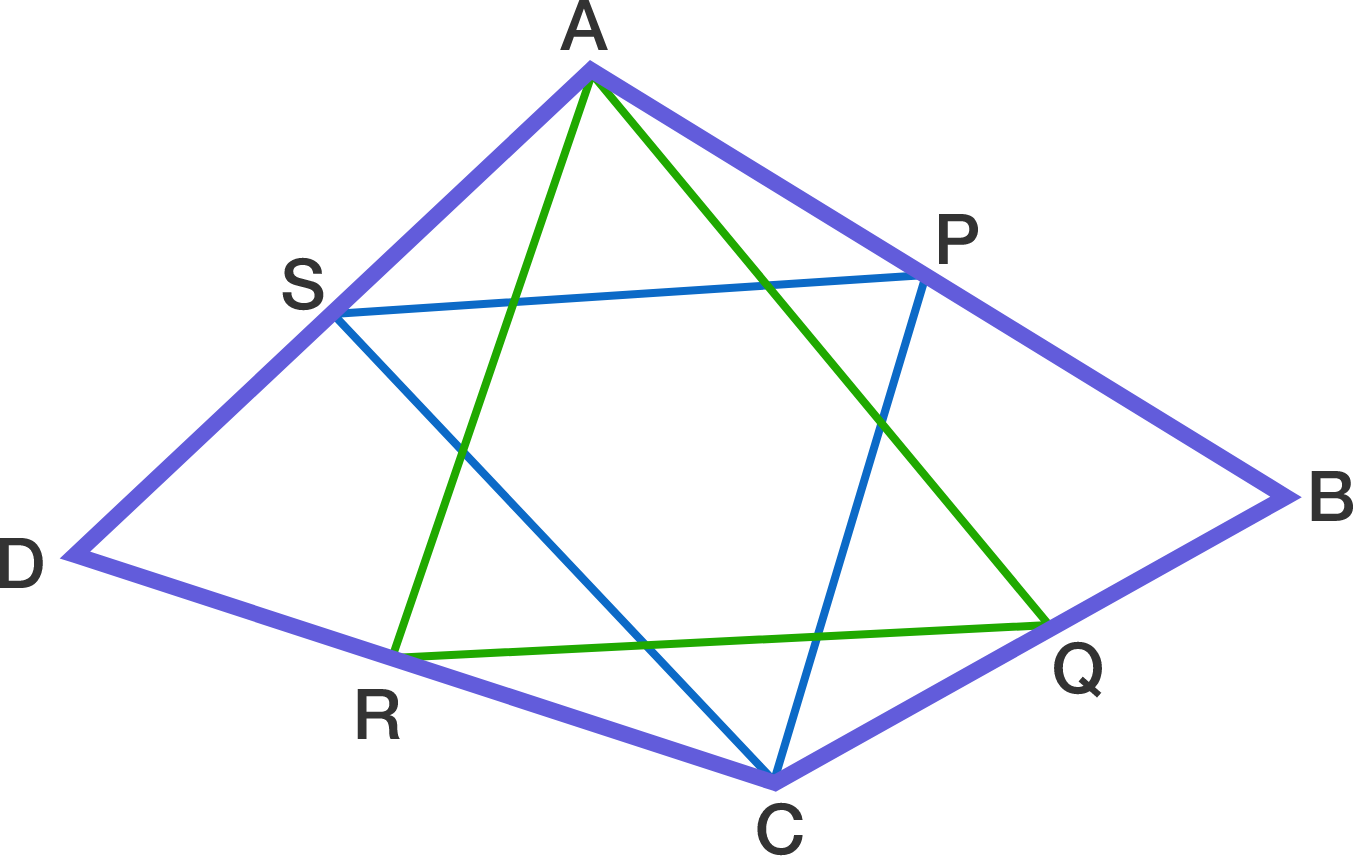# Just one step to regularity of quadrilateral

Geometry Level 3Let ABCD be a convex quadrilateral. Also let P, Q, R, S be the respective midpoints of sides AB, BC, CD, DA such that triangles AQR and CSP are both equilateral.

Find the largest angle (in degrees) of quadrilateral ABCD.

×